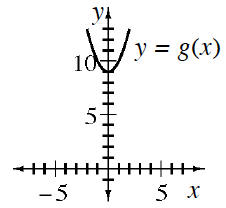### Home > INT2 > Chapter 10 > Lesson 10.1.3 > Problem10-44

10-44.The graph and table below represent two different quadratic functions.

 $x$ $-2$ $-1$ $0$ $1$ $2$ $f(x)$ $7$ $0$ $-3$ $-2$ $3$
1. Which function has a smaller minimum value? Explain how you know.

Are there any points given for $f(x)$ that are lower than those on $g(x)$?

$y = f(x)$; $f(0) = -3$ and $f(1) = -2$, both function values
are smaller than the minimum value of $g(0) = 9$.

2. Determine the roots of $g(x) = x^2 + 9$. Use your results to write the expression $x^2 + 9$ as a product.

Refer to problem 10-37 part (c) for additional help.